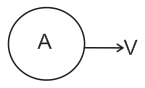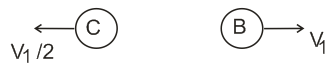# A nucleus A, with a finite de-broglie wavelength , undergoes spontaneous fission into nuclei B and C of equal mass. B files in the same direction as that of A, while C files in the opposite direction with a velocity equal to half of that of B. the de-broglie wavelengths  and  B and C are respectively:Option 1)Option 2)Option 3)Option 4)

Momentum convervation

initialAssume 'B' will move in same direction as of 'A' after fission

final\

So

as

Option 1)

Option 2)

Option 3)

Option 4)

Exams
Articles
Questions